# Our Blogs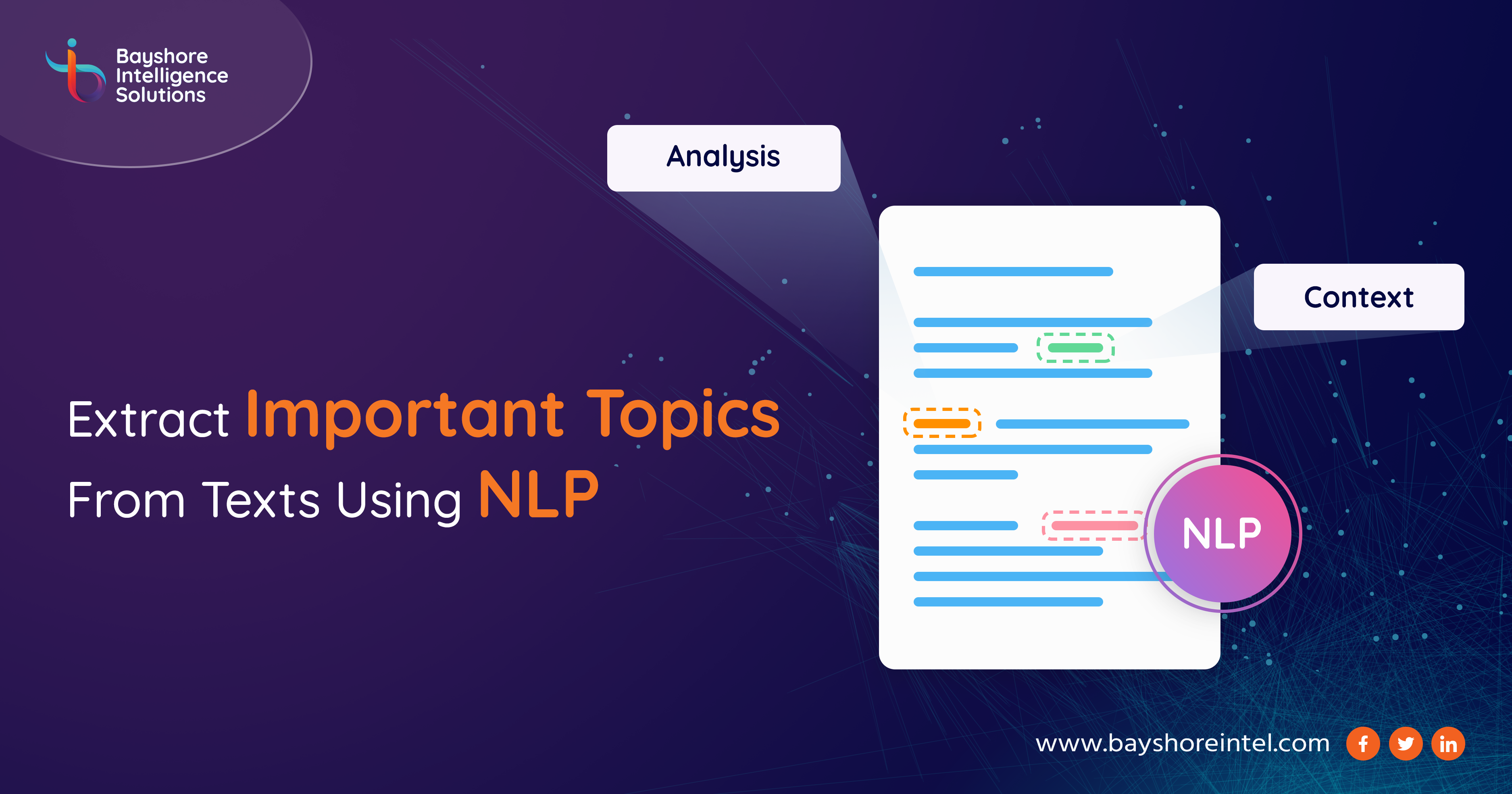23 November
2022### Introduction:

In our daily routine, sometimes we need to read various articles, news stories, advertisements, research papers, blogs, etc. To get a full understanding of that article or blog or research paper, we need to read the whole text.  But what happens when we need to read hundreds of articles or blogs or research papers? It will be hectic for an individual to do that. Let’s have an example of a Ph.D. scholar. Suppose he is researching a particular topic, and he needs to cite other research papers related to that topic. Now there are hundreds of papers available related to that topic and he must go through each of them to find the right papers. Hence, this will be a time-consuming tusk. In this blog, we are discussing a method with code (code will be discussed in python language), that will help an individual extract important topics from any text documents. Here the number of topics can also be varied according to individual requirements.

### Basic Understanding of Topics extraction:

Topic extraction is the automated process of extracting the words and phrases that are most relevant to an input text. Different methods are there for extracting topics from text.

Already there are two easy-to-use packages named RAKE (based on the Rapid Automatic Keyword Extraction algorithm) and YAKE (Yet another keyword extractor). However, these models typically work based on the statistical properties of a text and not so much on semantic similarity.

Hence, we will look into another method, where we will keep this semantic similarity in our mind. In this method, we are going to use BERT (Bidirectional Transformer), more precisely KeyBERT (a minimal and easy-to-use keyword extraction technique that leverages BERT embeddings)

However, the main topic of this article will not be the use of KeyBERT but a tutorial on how to use BERT to create your own keyword extraction model

### Step-by-Step Procedure:

1. Collect the text: First, we need to collect the text. We are having an example text of a research paper summary.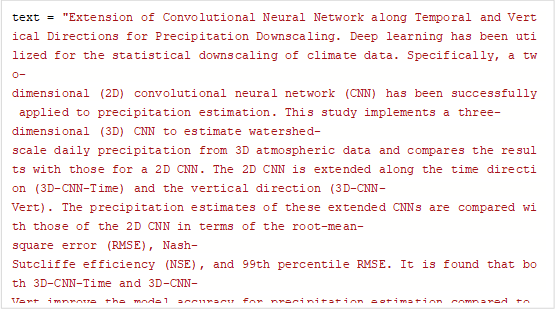2.  Extracting keywords:  Now we will extract keywords from the above text. We will remove the stop words followed by encoding each keyword with Bag of Words (considering only bi-gram and tri-gram).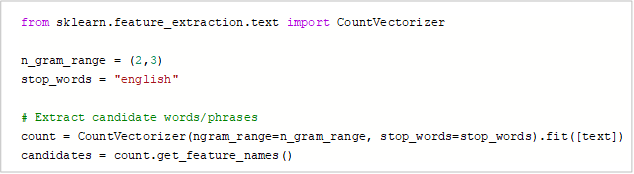The variable candidates are nothing but a list of each keyword.

3. Embeddings: Next, we will embed the text as well as the candidate keywords using BERT. In this case, we will use sentence transformers to encode both.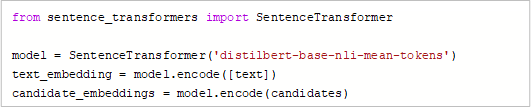Note: For large documents, there may be some token limit issue, in that case, we have to split the text into small pieces.

4. Distance calculation: Now we are having text embeddings as well as candidate embeddings. Now we must calculate the distance between each candidate with the text.

Here we will use cosine similarity to calculate the distance.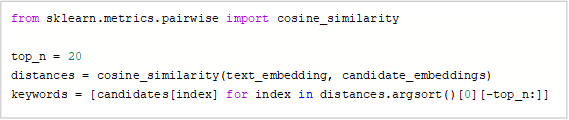Here we are taking the top 20 keywords based on distance score.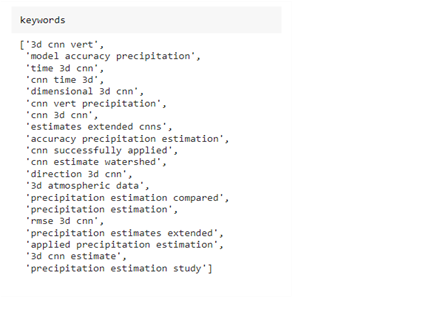Finally, we have extracted the important topics. Since we have used bi-grams and tri-grams, we can see the topics consisting of both two and three words.

So, by increasing the n-grams, we can take more words in each topic.

### Tuning the Result:

As we can see, the keywords are very similar to each other. In order to make it more diversified, we need to perform some more techniques on top of this.

Here we will perform below two techniques to diversify the keywords (any one of the below techniques can be used)

1. Max Sum Similarity (MSS)
2. Maximal Marginal Relevance (MMR)

Max Sum Similarity (MSS):

The maximum sum distance between pairs of data is defined as the pairs of data for which the distance between them is maximized.

In our case, we want to maximize the candidate’s similarity to the text while minimizing the similarity between candidates.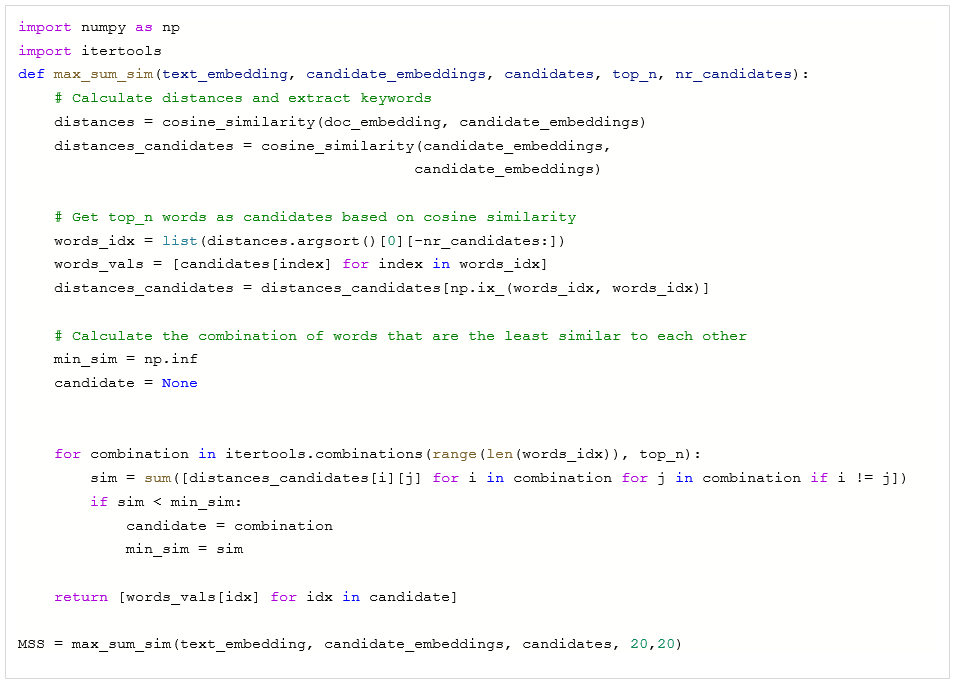Here we are creating a function for MSS to return the top 20 keywords.

Now, we can tune the nr_candidate parameter. Higher the nr_candidates value, the more diversity. We can compare the output below.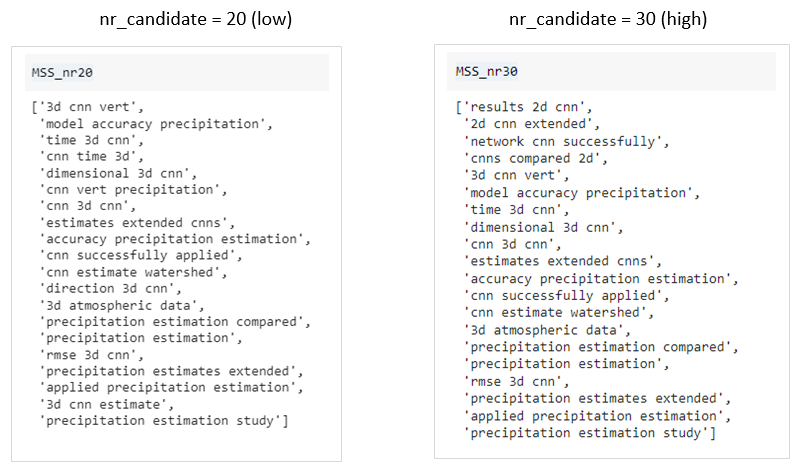As we can see from the above two results, the output coming from the function with nr_candidates = 20 is very similar to that coming from the vanilla cosine similarity, while the output coming from the function with nr_candiodates= 30 diversified the result.

So, we can tune this parameter as per the requirements

Maximal Marginal Relevance (MMR):

MMR tries to reduce the redundancy of results while at the same time maintaining query relevance of results for already ranked documents/phrases etc. Fortunately, a keyword extraction algorithm called EmbedRank has implemented a version of MMR that allows us to use it for diversifying our keywords/key phrases.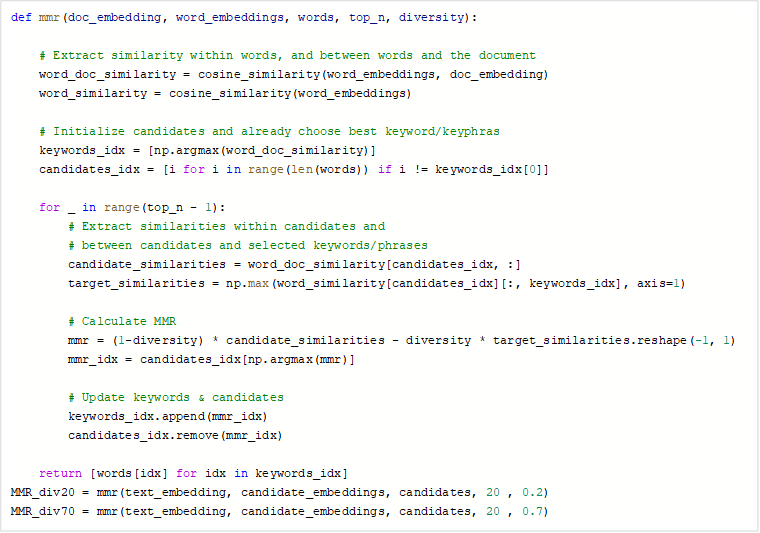Here also we can tune the diversity parameter. The higher the diversity value, the more will be the diversity in the result. We have used two values 20% and 70%. Diversity with 20% gives us a very similar result to that of the vanilla cosine similarity, while 70% gives some different results. From the below outputs, we can easily compare those two results.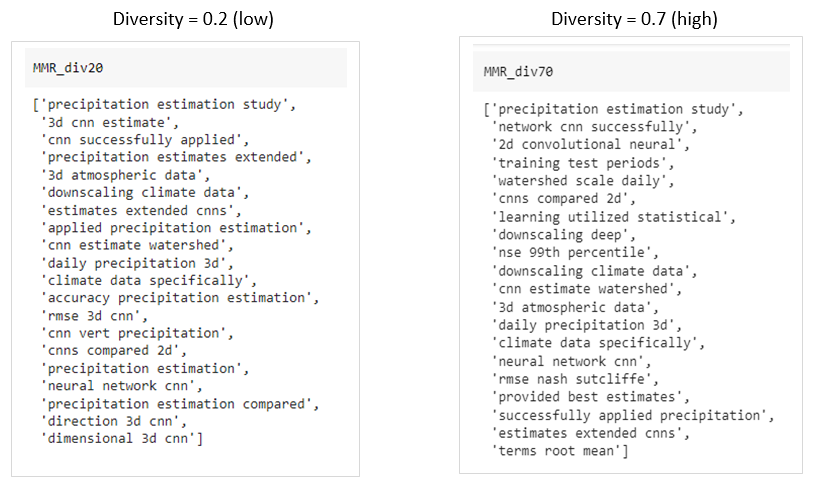As we can see, the two results are different, we can tune this parameter as per our requirements.

### Conclusion:

In this blog, I have tried to keep things as simple as possible. Here we can easily extract important topics from text.

We can add more layers on top of these results to filter the keywords. One such is to Verb removal (which means if any of the topics contain any verb, we can simply ignore that topic. It will give us a more genuine result).

There are a lot of use cases, where this technique can be used. One such use case I have tried to mention in this blog is Finding important topics from a summary of a research paper. It will help a researcher or reader to get a quick understanding of the topic of the paper.